PHY294H - Lecture35

Divergence theorem

The integral of the divergence of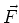over a volume, V, is equal to the integral of the component of the function through the surface, S, which encloses this volume. This is mathematically stated as,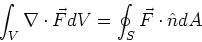(1)

Stokes theorem

The line integral of a vector function around a closed loop is equal to the curl of the function through any surface, S, which is connected to the closed loop.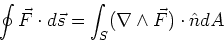(2)

Relation between current and current density

The relation we need is,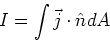(3)

Gauss' law for electric field

The Electric flux,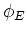, through a closed surface, S is equal to the net charge, q, enclosed within that surface.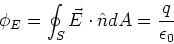(4)

Differential form,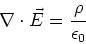(5)

Gauss's law for magnetic field

The Magnetic flux,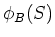, through a closed surface, S is equal to zero.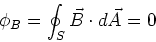(6)

Differential form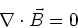(7)

Generalized Ampere's law

The path integral of the magnetic field around any closed loop, is equal to the current enclosed by the loop plus the displacement current enclosed by the loop.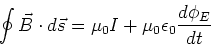(8)

Differential form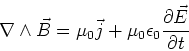(9)

The emf induced in a closed loop, is equal to the rate of change of the magnetic flux,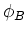, through the closed loop,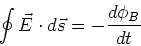(10)

Differential form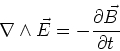(11)

The equations for EM waves in free space

In free space, there are no wires so the term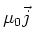is not needed. We want to combine Eq. (9) and (11). If we take a time derivative of Eq.(9) and then use Eq. (11), we find,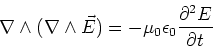(12)

If we take a time derivative of (11) then use Eq. (9) we find,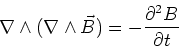(13)

An identity that is easy to prove (e.g. using Mathematica!) is,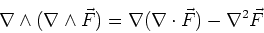(14)

Now note that in free space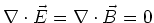, Using these expressions in Eqs. (12) and (13), we find,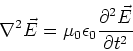(15)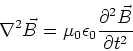(16)

These are both wave equations, which just means that they have solutions which are of the form,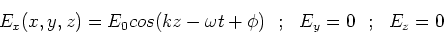(17)

This is an EM wave which travels in the z-directionand whose electric field oscillates in the x direction. Similar solutions exist for waves travelling in the x-direction and in the y-direction. Most of the physics can be deduced from this one component of the field. If we subsitute this expression into Eq.(15), we find,(18)

or, using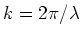,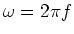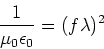(19)

This demonstrates that the velocity of the wave is,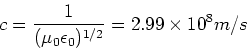(20)

This discovery is considered one of the most important of the 19th century and provides the unification of optics, electricity and magnetism. Since we have taken the Ey=Ez=0, we have,(21)

We also have,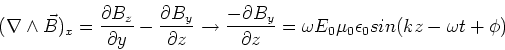(22)

If we integrate this expression with respect to z, we find,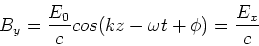(23)

This expression shows that the magnetic field is oscillating in phase with the electric field. The electric field oscillates in the x direction, the magnetic field oscillates in the y-direction and the wave travels in the z-direction.